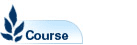Mathematics 2B - MATH2099

Faculty: Faculty of Science

Course Outline: http://www.maths.unsw.edu.au

Campus: Sydney

Units of Credit: 6

Indicative Contact Hours per Week: 6

Enrolment Requirements:

Prerequisite: MATH1231 or MATH1241 or MATH1251. Exclusions: MATH2501, MATH2601, and MATH2509

Excluded: BEES2041, BIOS2041, MATH2089, MATH2801, MATH2841, MATH2859, MATH2901

Tuition Fee: See Tuition Fee Schedule

Further Information: See Class Timetable

View course information for previous years.

Description

Linear algebra: Vector spaces, linear transformations, change of basis, inner products, orthogonalization, least squares approximation, QR factorization, determinants, eigenvalues and eigenvectors, diagonalization, Jordan forms, matrix exponentials and applications to systems of differential equations, other applications of linear algebra.
Probability and statistics: Sample spaces, probability, random variables and probability distributions, standard discrete and continuous distributions, multivariate distributions, Central Limit Theorem, statistical inference, confidence intervals and hypothesis testing, linear regression, inference in the linear model. Matlab will be used in this course.